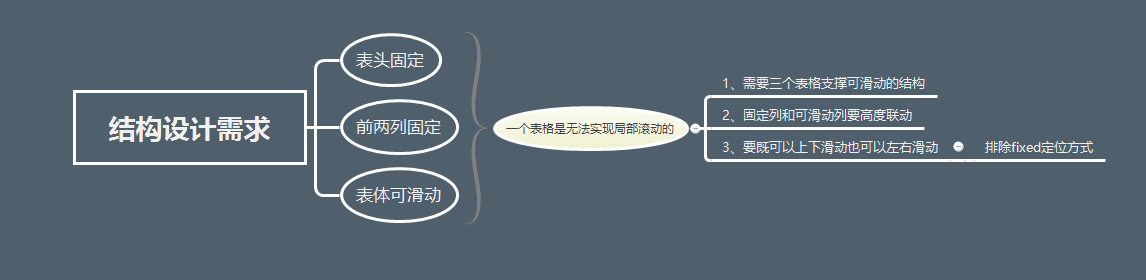通過js實現固定表格的表頭和某列

設計過程具體實現
1. 元素結構
var TableWrap = React.createClass({
render:function(){
var data = this.props.data;
return(
<div className='table-wrapper'>
<div className="table-body-wrapper">
<Tablefix className = 'table-fixded' data = {data} />
<Tablebody className='table-body' data = {data} />
</div>
</div>
)
}
})

2. 表格聯動
$(funciton autoWidth(){ for(var i = 1 ; i <=$('.tablebody tr').length ; i++){
var tableH = $(".tablebody tr:nth-child("+i+") td").outerHeight(true);$(".Tablefix tr:nth-child("+i+") td:last-child").css("height",tableH);
}
})

3. 定位設計
$(function fixed(){$(window).scroll(function () {
if($(this).scrollLeft()>0){ if($(this).scrollLeft()<60){ //60用於控制靈敏度，數值越小 靈敏度越高
$(".tablefix-wrapper").css("left","0px"); }else {$(".tablefix-wrapper").css("left",$(this).scrollLeft()); } } if($(this).scrollTop()>0){
if ($(this).scrollTop()<60) {$(".header-wrapper").css("top","0px");
}else {
$(".header-wrapper").css("top",$(this).scrollTop());
}
}
})
})

展示效果
1. 實現表頭上下固定，左右可以滾動
2. 實現固定列左右固定，上下可以滑動
3. 滾動條位於視窗，便於滑動